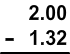# Making Change

Print Rate 0 stars Common Core
Lesson size:
Message preview:
Someone you know has shared lesson with you:

To play this lesson, click on the link below:

https://www.turtlediary.com/lesson/making-change.html

Hope you have a good experience with this site and recommend to your friends too.

Login to rate activities and track progress.
Login to rate activities and track progress.

Change is the amount of money you receive back when you make a purchase.

To find out how much change you should receive, subtract the price of the purchase from the amount of money you gave.

Remember, that when you subtract money, you have to line up the decimals. If there is no decimal, it is understood to be at the end of the number.

Let's take a look at some examples:

## Example 1

Annie bought a pack of pencils for \$1.32. She handed two dollars to the cashier. How much change will she receive?

We must simply subtract \$1.32 from two dollars. But, don't forget to line up the decimals.

Remember that two dollars can be written as \$2.00.Now simply subtract:Yes, it is reasonable that you would receive 68¢ back as change. (If we had put \$68 as our answer, we would realize it doesn't make sense to receive \$68 back, when you only gave the cashier \$2).

## Example 2

Annie bought a pack of pencils for \$1.32. She handed two dollars to the cashier. How much change will she receive?

We must start by finding out how much change she will receive.

Line up the decimals and subtract:But the question asks us what bills/coins she will receive. The \$1 of course would be a dollar bill.

But what about the 65 cents?

Cashiers typically give back the fewest coins possible. So, the cashier would give two quarters (50 cents), a dime (10 cents), and a nickel (5 cents).

Answer: 1 dollar, 2 quarters, 1 dime, 1 nickel

### Example 3

Camden is selling candy bars for \$1.25 each to raise money for a school fundraiser. If you buy one and pay him with a \$5 bill, what bills/coins should he give you back as change?

First, you must subtract to find the amount of change. Remember to line up the decimals.

\$5 doesn't appear to have a decimal, so it is at the end, and we can also fill in the zeros after the decimal: \$5.00What bills/coins would this be?

Of course, \$3 is 3 dollar bills.

And 75 cents is 3 quarters (25 cents each).

Answer: 3 dollar bills, 3 quarters

## Making Change

• Change is the amount of money you receive back when you make a purchase.
• To make change, subtract the price of the purchase from the amount of money given.
• Remember, that when you subtract money, you have to line up the decimals. If there is no decimal, it is understood to be at the end of the number.

## Similar Lessons

Become premium member to get unlimited access.